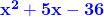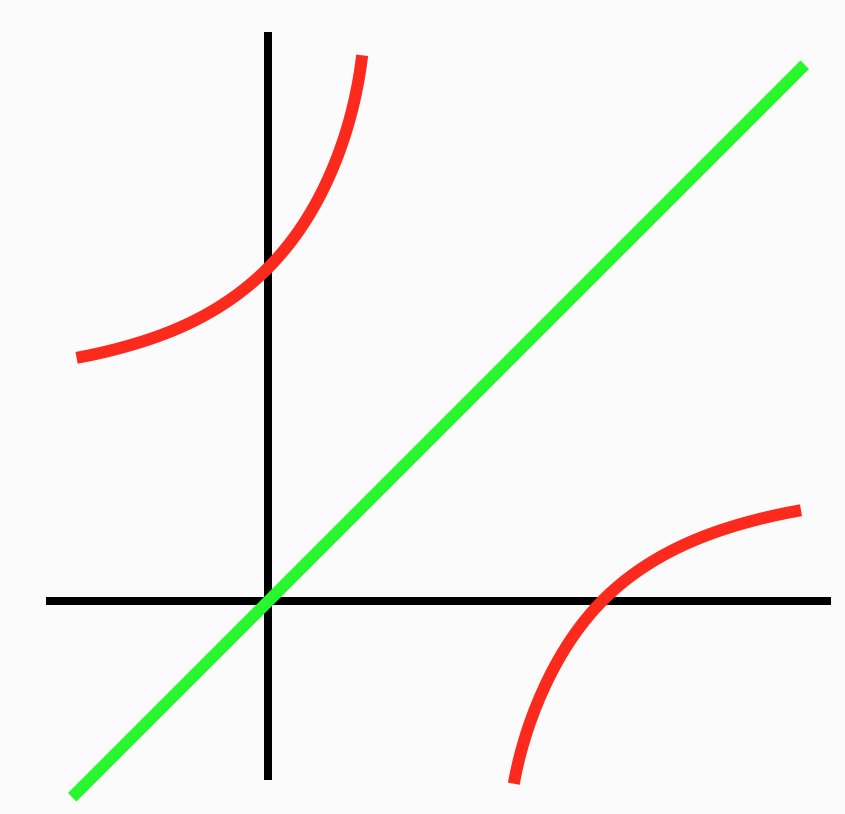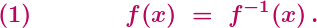## Polynomially Perverse

What, with its stupid curriculastupid texts and really monumentally stupid exams, it’s difficult to imagine a wealthy Western country with worse mathematics education than Australia. Which is why God gave us New Zealand.

Earlier this year we wrote about the first question on New Zealand’s 2016 Level 1 algebra exam:

A rectangle has an area of. What are the lengths of the sides of the rectangle in terms of.

Obviously, the expectation was for the students to declare the side lengths to be the linear factors x – 4 and x + 9, and just as obviously this is mathematical crap. (Just to hammer the point, set x = 5, giving an area of 14, and think about what the side lengths “must” be.)

One might hope that, having inflicted this mathematical garbage on a nation of students, the New Zealand Qualifications Authority would have been gently slapped around by a mathematician or two, and that the error would not be repeated. One might hope this, but, in these idiot times, it would be very foolish to expect it.

A few weeks ago, New Zealand maths education was in the news (again). There was lots of whining about “disastrous” exams, with “impossible” questions, culminating in a pompous petition, and ministerial strutting and general hand-wringing. Most of the complaints, however, appear to be pretty trivial; sure, the exams were clunky in certain ways, but nothing that we could find was overly awful, and nothing that warranted the subsequent calls for blood.

What makes this recent whining so funny is the comparison with the deafening silence in September. That’s when the 2017 Level 1 Algebra Exams appeared, containing the exact same rectangle crap as in 2016 (Question 3(a)(i) and Question 2(a)(i)). And, as in 2016, there is no evidence that anyone in New Zealand had the slightest concern.

People like to make fun of all the sheep in New Zealand, but there’s many more sheep there than anyone suspects.

UPDATE (04/02/19): An Oxford school text joins in the fun.Our sixth and final post on the 2017 VCE exam madness is on some recurring nonsense in Mathematical Methods. The post will be relatively brief, since a proper critique of every instance of the nonsense would be painfully long, and since we’ve said it all before.

The mathematical problem concerns, for a given function f, finding the solutions to the equationThis problem appeared, in various contexts, on last month’s Exam 2 in 2017 (Section B, Questions 4(c) and 4(i)), on the Northern Hemisphere Exam 1 in 2017 (Questions 8(b) and 8(c)), on Exam 2 in 2011 (Section 2, Question 3(c)(ii)), and on Exam 2 in 2010 (Section 2, Question 1(a)(iii)).

Unfortunately, the technique presented in the three Examiners’ Reports for solving equation (1) is fundamentally wrong. (The Reports are here, here and here.) In synch with this wrongness, the standard textbook considers four misleading examples, and its treatment of the examples is infused with wrongness (Chapter 1F). It’s a safe bet that the forthcoming Report on the 2017 Methods Exam 2 will be plenty wrong.

What is the promoted technique? It is to ignore the difficult equation above, and to solve instead the presumably simpler equationor perhaps the equationWhich is wrong.

It is simply not valid to assume that either equation (2) or (2)’ is equivalent to (1). Yes, as long as the inverse of f exists then equation (2)’ is equivalent to equation (2): a solution x to (2)’ will also be a solution to (2), and vice versa. And, yes, then any solution to (2) and (2)’ will also be a solution to (1). The converse, however, is in general false: a solution to (1) need not be a solution to (2) or (2)’.

It is easy to come up with functions illustrating this, or think about the graph above, or look here.

OK, the VCAA might argue that the exams (and, except for a couple of up-in-the-attic exercises, the textbook) are always concerned with functions for which solving (2) or (2)’ happens to suffice, so what’s the problem? The problem is that this argument would be idiotic.

Suppose that we taught students that roots of polynomials are always integers, instructed the students to only check for integer solutions, and then carefully arranged for the students to only encounter polynomials with integer solutions. Clearly, that would be mathematical and pedagogical crap. The treatment of equation (1) in Methods exams, and the close to universal treatment in Methods more generally, is identical.

OK, the VCAA might continue to argue that the students have their (stupifying) CAS machines at hand, and that the graphs of the particular functions under consideration make clear that solving (2) or (2)’ suffices. There would then be three responses:

(i) No one tests whether Methods students do anything like a graphical check, or anything whatsoever.

(ii) Hardly any Methods students do do anything. The overwhelming majority of students treat equations (1), (2) and (2)’ as automatically equivalent, and they have been given explicit license by the Examiners’ Reports to do so. Teachers know this and the VCAA knows this, and any claim otherwise is a blatant lie. And, for any reader still in doubt about what Methods students actually do, here’s a thought experiment: imagine the 2018 Methods exam requires students to solve equation (1) for the function f(x) = (x-2)/(x-1), and then imagine the consequences.

(iii) Even if students were implicitly or explicitly arguing from CAS graphics, “Look at the picture” is an absurdly impoverished way to think about or to teach mathematics, or pretty much anything. The power of mathematics is to be able take the intuition and to either demonstrate what appears to be true, or demonstrate that the intuition is misleading. Wise people are wary of the treachery of images; the VCAA, alas, promotes it.

The real irony and idiocy of this situation is that, with natural conditions on the function f, equation (1) is equivalent to equations (2) and (2)’, and that it is well within reach of Methods students to prove this. If, for example, f is a strictly increasing function then it can readily be proved that the three equations are equivalent. Working through and applying such results would make for excellent lessons and excellent exam questions.

Instead, what we have is crap. Every year, year after year, thousands of Methods students are being taught and are being tested on mathematical crap.